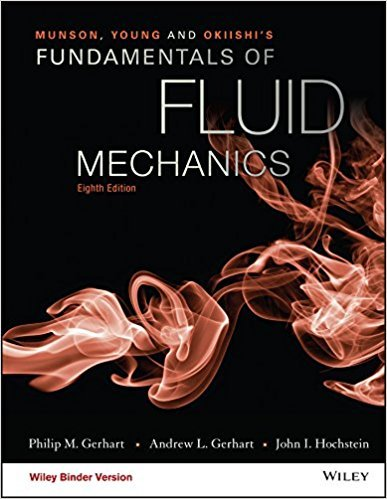×
×

# Water flows from a nozzle with a speed of V = 10 m/s and iscollected in a container thatISBN: 9781119080701 456

## Solution for problem 4.69 Chapter 4.4

Fundamentals of Fluid Mechanics | 8th Edition

• Textbook Solutions
• 2901 Step-by-step solutions solved by professors and subject experts
• Get 24/7 help from StudySoup virtual teaching assistantsFundamentals of Fluid Mechanics | 8th Edition

4 5 1 331 Reviews
21
4
Problem 4.69

Water flows from a nozzle with a speed of V = 10 m/s and iscollected in a container that moves toward the nozzle with a speedof Vcv = 2 m/s as shown in Fig. P4.69. The moving control surfaceconsists of the inner surface of the container. The system consistsof the water in the container at time t = 0 and the water betweenthe nozzle and the tank in the constant diameter stream at t = 0. Attime t = 0.1 s what volume of the system remains outside of thecontrol volume? How much water has entered the control volumeduring this time period? Repeat the problem for t = 0.3 s.

Step-by-Step Solution:
Step 1 of 3

Some basic physics – Kepler’s laws seem kind of arbitrary – there are basic laws of nature and physics that underlie Kepler’s laws Motion Position Requires a coordinate system (ex: it lies 3 meters above …..) Displacement – change...

Step 2 of 3

Step 3 of 3

##### ISBN: 9781119080701

The answer to “Water flows from a nozzle with a speed of V = 10 m/s and iscollected in a container that moves toward the nozzle with a speedof Vcv = 2 m/s as shown in Fig. P4.69. The moving control surfaceconsists of the inner surface of the container. The system consistsof the water in the container at time t = 0 and the water betweenthe nozzle and the tank in the constant diameter stream at t = 0. Attime t = 0.1 s what volume of the system remains outside of thecontrol volume? How much water has entered the control volumeduring this time period? Repeat the problem for t = 0.3 s.” is broken down into a number of easy to follow steps, and 110 words. This full solution covers the following key subjects: . This expansive textbook survival guide covers 112 chapters, and 1357 solutions. Fundamentals of Fluid Mechanics was written by and is associated to the ISBN: 9781119080701. This textbook survival guide was created for the textbook: Fundamentals of Fluid Mechanics, edition: 8. The full step-by-step solution to problem: 4.69 from chapter: 4.4 was answered by , our top Science solution expert on 03/16/18, 03:21PM. Since the solution to 4.69 from 4.4 chapter was answered, more than 212 students have viewed the full step-by-step answer.

Unlock Textbook Solution